# Geometric Graphing Functions: Tutoring Solution Chapter Exam

Exam Instructions:

Choose your answers to the questions and click 'Next' to see the next set of questions. You can skip questions if you would like and come back to them later with the yellow "Go To First Skipped Question" button. When you have completed the practice exam, a green submit button will appear. Click it to see your results. Good luck!

Answered 0 of 30

### Page 2

#### Question 6 6. In the pictured graph of f(x) = x^2 + 6x, for which values of x is the function increasing?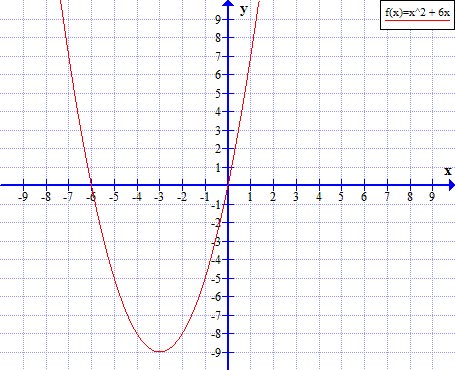#### Question 7 7. Which of the following shows the graph of the equation below?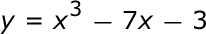#### Question 9 9. Which of the following shows the graph of the equation below?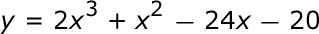#### Question 10 10. What is the equation of the graph below?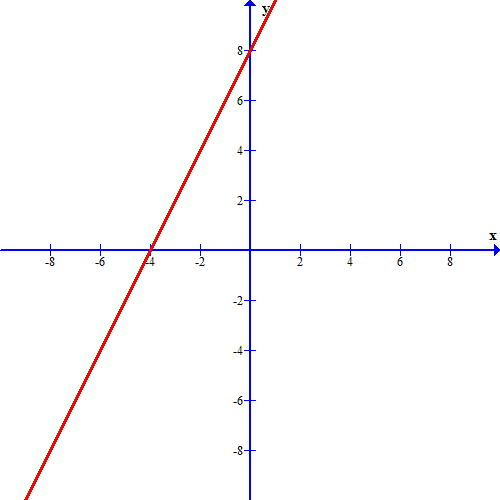### Page 3

#### Question 11 11. In the pictured graph of f(x) = x^2 - 4x, for which values of x is f(x) > 0?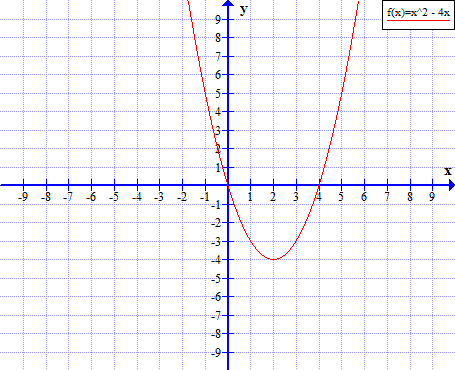#### Question 14 14. Which of the following shows the graph of the equation below?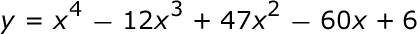### Page 4

#### Question 20 20. In the pictured graph of f(x) = -2x^2 + 4x - 2, for which values of x is the function decreasing?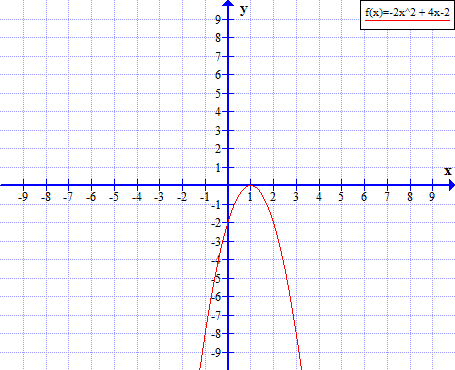### Page 6

#### Geometric Graphing Functions: Tutoring Solution Chapter Exam Instructions

Choose your answers to the questions and click 'Next' to see the next set of questions. You can skip questions if you would like and come back to them later with the yellow "Go To First Skipped Question" button. When you have completed the practice exam, a green submit button will appear. Click it to see your results. Good luck!

Support